### 10.3.  Displace

Revision History
Revision \$Revision: 2567 \$ 2007-07-23 ude

#### 10.3.1.  Overview

Figure 16.202.  Displacement examples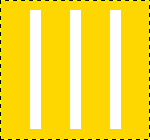Original image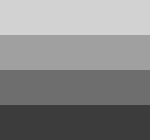The displacement map has four grey stripes with values of 210, 160, 110, and 60, respectively.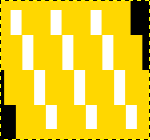X displacement coefficient is 30. Vacated pixels are black. The image areas corresponding to light gray (≥128) were displaced 19 and 8 pixels to the left. The image areas corresponding to dark gray (≤127) were displaced 4 and 15 pixels to the right.

This filter uses a “displace-map” to displace corresponding pixels of the image. This filter displaces the content of the specified drawable (active layer or selection) by the amounts specified in X and Y Displacement multiplied by the intensity of the corresponding pixel in the 'displace map' drawables. Both X and Y displace maps should be gray-scale images and have the same size as the drawable . This filter allows interesting distortion effects.

#### 10.3.2.  Activate the filter

You can find this filter through FiltersMapDisplace

#### 10.3.3.  Options (General)

Figure 16.203.  Displace filter options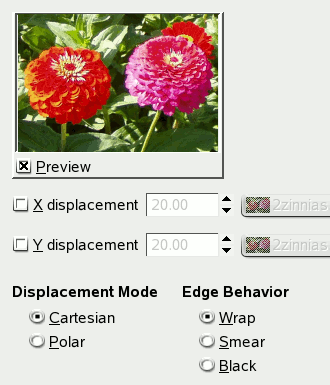Preview

Uncheck this option if your processor is slow.

Displacement Mode

You can choose working in cartesian coordinates, where pixels are displaced in X or Y direction, or working in polar coordinates, where the image is pinched and whirled by displacing pixels in radial or tangent direction.

On Edges

These options allows you to set displacement behaviour on active layer or selection edges:

• Wrap : With this option, what disappears on one edge reappears on the opposite edge.

• Smear : With this option, pixels vacated by displacement are replaced with pixels stretched from the adjacent part of the image.

• Black : With this option, pixels vacated by displacement are replaced with black.

##### 10.3.3.1.  Cartesian Displacement Mode

Figure 16.204.  Displace filter options (Cartesian)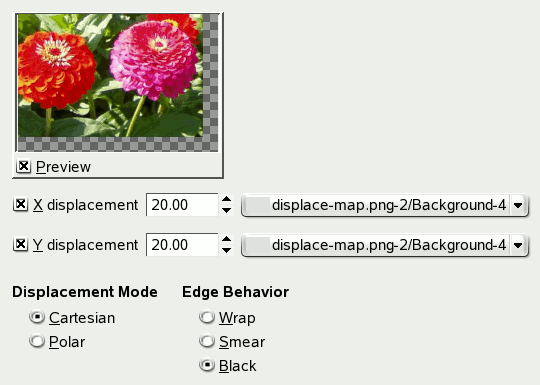Displacement Mode: Cartesian

In both modes, direction and amount of displacement depend on the intensity of the corresponding pixel in the displacement map.

The map, that should be a grayscale image, has 256 gray levels (0-255), the (theoretical) average value is 127.5. The filter displaces image pixels corresponding to pixels with values less than 127.5 (0 to 127) in map to one direction, corresponding to pixels with values from 128 to 255 to the opposite direction.

X/Y Displacements

If the respective option is activated, image pixels corresponding to pixels from 0 to 127 will be displaced to the right for X, downwards for Y, image pixels corresponding to pixels from 128 to 255 will be displaced to the left for X, upwards for Y.

What you enter in input boxes, directly or by using arrow-head buttons, is not the actual displacement. It's a coefficient used in a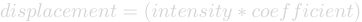formula, which gives the pixel actual displacement according to the scaled intensity  of the corresponding pixel in map, modulated by the coefficient you enter. Introducing intensity into formula is important: this allows progressive displacement by using a gradient map.

This value may be positive or negative. A negative displacement is reverse of a positive one. The value varies in limits equal to the double of image dimensions.

When you click on the drop-down list button, a list appears where you can select a displacement map. To be present in this list, an image must respect two conditions. First, this image must be present on your screen when you call filter. Then, this image must have the same dimensions as the original image. Most often, it will be a duplicate original image, which is transformed to grey scale and modified appropriately, with a gradient. It may be possible to use RGB images, but color luminosity is used making result prevision difficult. Map may be different in horizontal and vertical directions.

##### 10.3.3.2.  Polar Displacement Mode

Figure 16.205.  Displace filter options (Polar)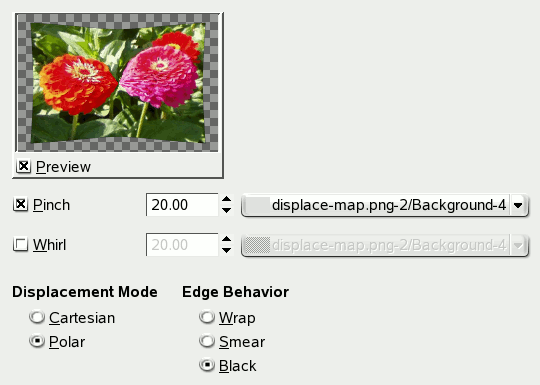Displacement Mode: Polar

Pinch

If this option is activated, the radial coordinates (i.e. the distance to the image's midpoint, the “pole”) of the pixels will be changed. Image pixels corresponding to map pixels from 0 to 127 will be displaced outwards, image pixels corresponding to pixels from 128 to 255 will be displaced towards center.

For the values and the displacement map see above (“X/Y Displacements”).

The displacement is independent from the polar distance, all pixels are displaced by the same amount. So the image will not only be stretched or compressed, but also distorted: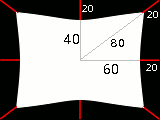Image distortion by Pinch option

A 160x120 pixel image, plain white displacement map, and displacement coefficient 20.0: this results in a 20 pixels displacement towards center. This is a horizontal reduction in size by 25%, vertical by 33%, and diagonal by 20%, so the image will be distorted.

Whirl

If this option is activated, the angular coordinates of the image pixels will be “displaced” by a map pixel dependent amount. For a plain displacement map, the image will be rotated, otherwise it will be whirled.

Image pixels corresponding to pixels from 0 to 127 in the map will be displaced counterclockwise, image pixels corresponding to pixels from 128 to 255 will be displaced clockwise.

For the values and the displacement map see above.

NoteFor a plain, non neutral map, if displace mode “Polar” is enabled, this filter works like Whirl and Pinch.

#### 10.3.4.  Using gradient to bend a text

2. Duplicate this image. Activate this duplicate and make it gray-scaled ( ImageModeGrayScale ). Fill it with the wanted gradient. This image will be your Displacement map, with the dimensions of original image.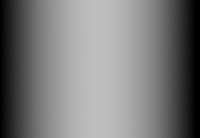3. Activate original image. Create a Text Layer with your text. Set layer to image size: right-click on the layer in layer dialog and, in the pop-menu, click on “Layer to image size”. Note that letters in text layer lie on a transparent background; now this filter doesn't displace transparent pixels. Only letters will be displaced.4. Activate the text layer. Open the Displace filter window. Set parameters, particularly the displacement coefficient, according to the result in Preview. Click OK.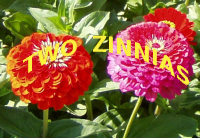This method also applies to standard layers: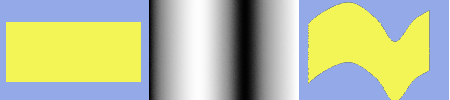Tip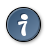To get the wanted gradient, first draw a black to white gradient. Then use the Curves tool to modify the gradient curve.

#### 10.3.5.  Displacement Calculation

The following section will show you how to calculate the amount of displacement, if you are interested in these details. If you don't want to know it, you can safely omit this section.

The overview example showed the X displacement using a coefficient of 30.0: 19, 8, 4, or 15 pixels, depending on the grey level of the displacement map's color.

Why just these amounts? That's easy: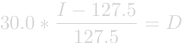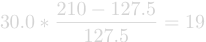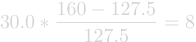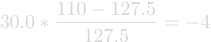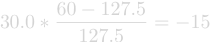If you check these equations, you will notice that the values they give are not exactly the results we retained in the example (using non-integers, that's not surprising). So, were the results rounded to the nearest integer and then the pixels were displaced by a whole-numbered amount? No. Every pixel is displaced exactly by the calculated amount; a “displacement by a fractional amount” is realized by interpolation. A closer look at the example image will show it:

Figure 16.206.  A closer look at the displacement example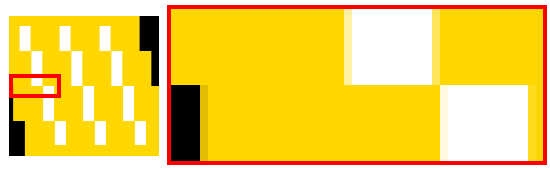A small area zoomed in by 800 percent.

The displacement causes small (one pixel wide) areas of intermediate colors at the edges of plain color areas. E.g., the black area (zoomed in image) is caused by a displacement of -4.12, so the intermediate color is 12% black and 88% gold.

So if you select a displacement coefficient of 30.01 instead of 30.00, you will indeed get a different image, although you won't see the difference, of course.

 Scaled intensity = (intensity - 127.5) / 127.5; see Section 10.3.5, “ Displacement Calculation.